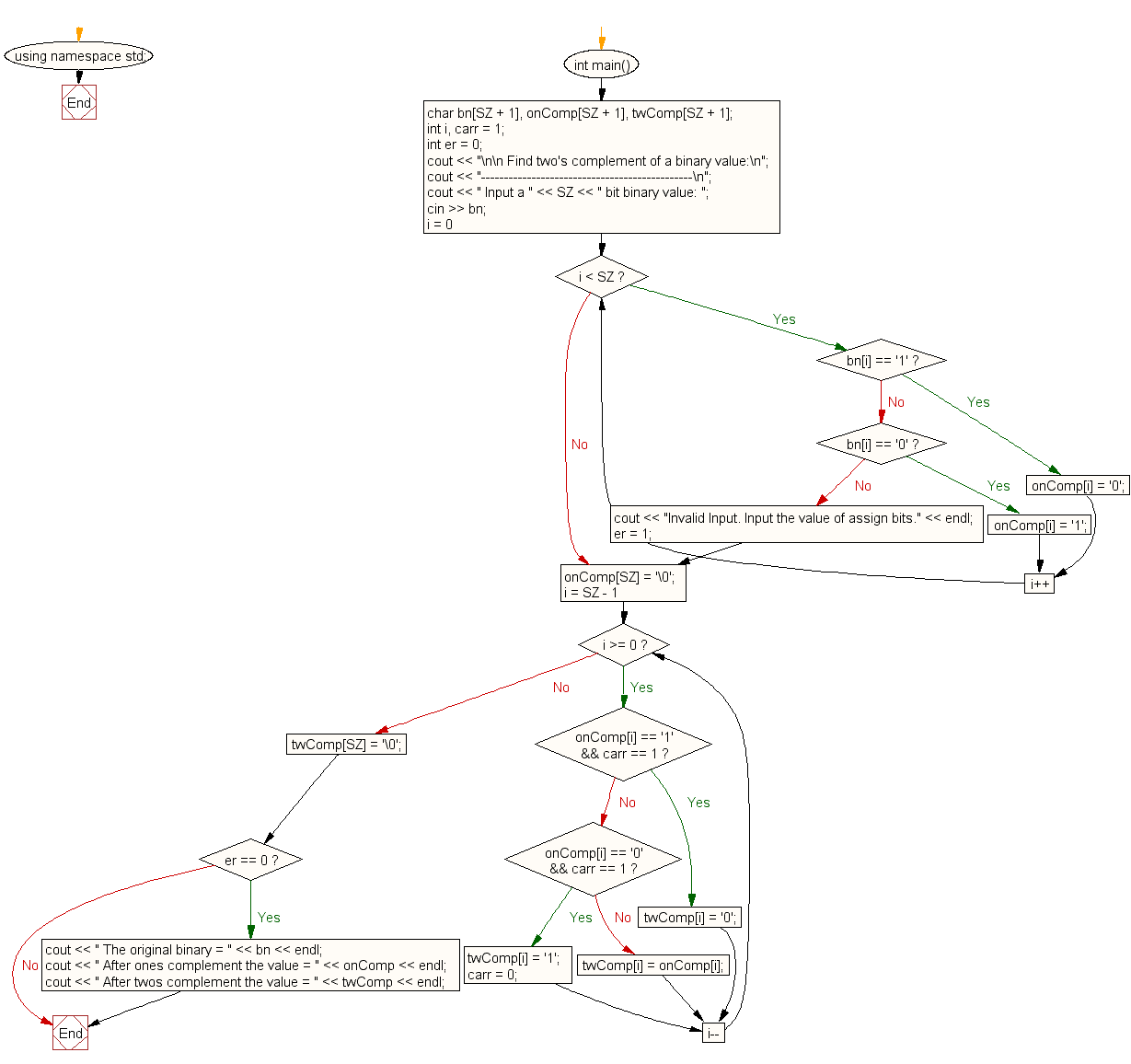﻿ C++ Exercises: Find two's complement of a binary number - w3resource# C++ Exercises: Find two's complement of a binary number

## C++ For Loop: Exercise-65 with Solution

Write a program in C++ to find two's complement of a binary number.

Sample Solution:-

C++ Code :

``````#include <iostream>
#define SZ 8
using namespace std;
int main()
{
char bn[SZ + 1], onComp[SZ + 1], twComp[SZ + 1];
int i, carr = 1;
int er = 0;
cout << "\n\n Find two's complement of a binary value:\n";
cout << "----------------------------------------------\n";
cout << " Input a " << SZ << " bit binary value: ";
cin >> bn;
for (i = 0; i < SZ; i++)
{
if (bn[i] == '1')
{
onComp[i] = '0';
}
else if (bn[i] == '0')
{
onComp[i] = '1';
}
else
{
cout << "Invalid Input. Input the value of assign bits." << endl;
er = 1;
break;
}
}
onComp[SZ] = '\0';

for (i = SZ - 1; i >= 0; i--)
{
if (onComp[i] == '1' && carr == 1)
{
twComp[i] = '0';
}
else if (onComp[i] == '0' && carr == 1)
{
twComp[i] = '1';
carr = 0;
}
else
{
twComp[i] = onComp[i];
}
}
twComp[SZ] = '\0';
if (er == 0)
{
cout << " The original binary = " << bn << endl;
cout << " After ones complement the value = " << onComp << endl;
cout << " After twos complement the value = " << twComp << endl;
}
}
``````

Sample Output:

``` Find two's complement of a binary value:
----------------------------------------------
Input a 8 bit binary value: 01101110
The original binary = 01101110
After ones complement the value = 10010001
After twos complement the value = 10010010
```

Flowchart:C++ Code Editor:

Contribute your code and comments through Disqus.

What is the difficulty level of this exercise?

﻿